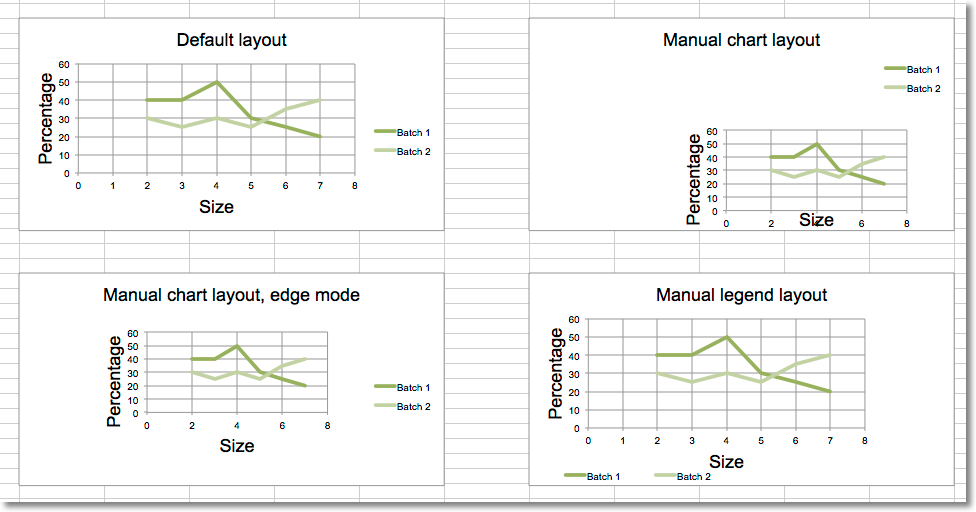# Changing the layout of plot area and legend¶

The layout of the chart within the canvas can be set by using the layout property of an instance of a layout class.

## Chart layout¶

### Size and position¶

The chart can be positioned within its container. `x` and `y` adjust position, `w` and `h` adjust the size . The units are proportions of the container. A chart cannot be positioned outside of its container and the width and height are the dominant constraints: if x + w > 1, then x = 1 - w.

x is the horizontal position from the left
y is the vertical position from the top
h is the height of the chart relative to its container
w is the width of the box

### Mode¶

In addition to the size and position, the mode for the relevant attribute can also be set to either factor or edge. Factor is the default:

```layout.xMode = edge
```

### Target¶

The layoutTarget can be set to `outer` or `inner`. The default is `outer`:

```layout.layoutTarget = inner
```

## Legend layout¶

The position of the legend can be controlled either by setting its position: `r`, `l`, `t`, `b`, and `tr`, for right, left, top, bottom and top right respectively. The default is `r`.

```legend.position = 'tr'
```

or applying a manual layout:

```legend.layout = ManualLayout()
```
```from openpyxl import Workbook, load_workbook
from openpyxl.chart import ScatterChart, Series, Reference
from openpyxl.chart.layout import Layout, ManualLayout

wb = Workbook()
ws = wb.active

rows = [
['Size', 'Batch 1', 'Batch 2'],
[2, 40, 30],
[3, 40, 25],
[4, 50, 30],
[5, 30, 25],
[6, 25, 35],
[7, 20, 40],
]

for row in rows:
ws.append(row)

ch1 = ScatterChart()
xvalues = Reference(ws, min_col=1, min_row=2, max_row=7)
for i in range(2, 4):
values = Reference(ws, min_col=i, min_row=1, max_row=7)
series = Series(values, xvalues, title_from_data=True)
ch1.series.append(series)

ch1.title = "Default layout"
ch1.style = 13
ch1.x_axis.title = 'Size'
ch1.y_axis.title = 'Percentage'
ch1.legend.position = 'r'

from copy import deepcopy

# Half-size chart, bottom right
ch2 = deepcopy(ch1)
ch2.title = "Manual chart layout"
ch2.legend.position = "tr"
ch2.layout=Layout(
manualLayout=ManualLayout(
x=0.25, y=0.25,
h=0.5, w=0.5,
)
)

# Half-size chart, centred
ch3 = deepcopy(ch1)
ch3.layout = Layout(
ManualLayout(
x=0.25, y=0.25,
h=0.5, w=0.5,
xMode="edge",
yMode="edge",
)
)
ch3.title = "Manual chart layout, edge mode"

# Manually position the legend bottom left
ch4 = deepcopy(ch1)
ch4.title = "Manual legend layout"
ch4.legend.layout = Layout(
manualLayout=ManualLayout(
yMode='edge',
xMode='edge',
x=0, y=0.9,
h=0.1, w=0.5
)
)